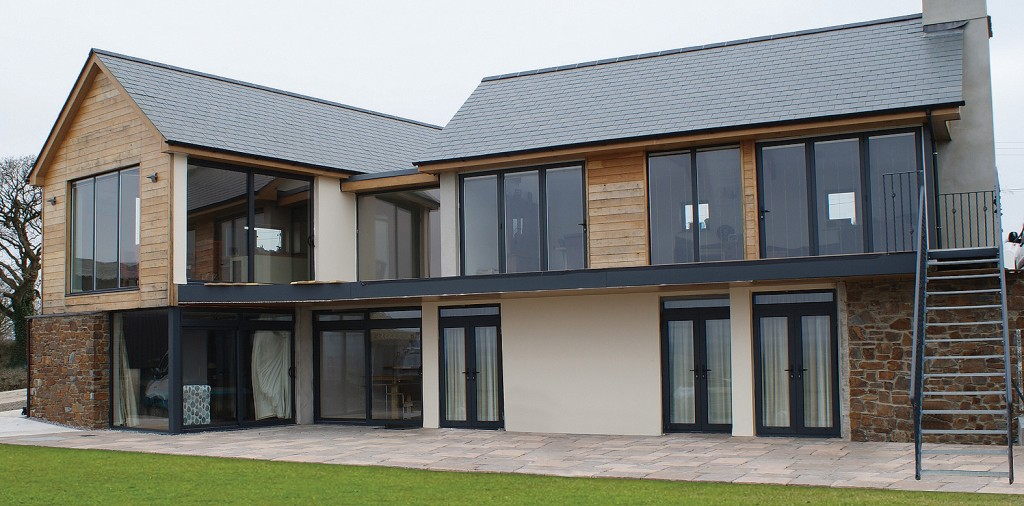## Restore Window Frames

#### How to calculate which size kit you will need

Simply measue all the sides of your window and the multipy by the width of the frame to calculate the total area.

Example

a=1m

b=2m

c=1m

d=1m

Total Length 5m

e= 0.2m or 20cm Width

1000 x 0.2 = 1m2

KIT COVERAGE

Small KIT      =    2.5 m2

Medium Kit  =   5     m2

Large KIt       =   12   m2

Try Our Excel

Calculator

HERE